Custom Legends with EarthPy

Learn how to create discrete legends for raster plots with classes that you define in Python using EarthPy.

Plotting Data in Python Using EarthPy

Note

The examples below will show you how to use the draw_legend() function for creating plots

Plot Continuous Data

Let’s explore a simple plot using EarthPy. To begin, import the needed packages and create an array to be plotted. Below we plot the data as continuous with a colorbar using the plot_bands() function.

import matplotlib.pyplot as plt
import earthpy.plot as ep
import numpy as np

# Create a numpy array. Let's pretend this is what you want to plot.
arr = np.random.randint(4, size=(5, 5))

# When plot_bands is updated a cbar will be here as well
ep.plot_bands(arr)
plt.show()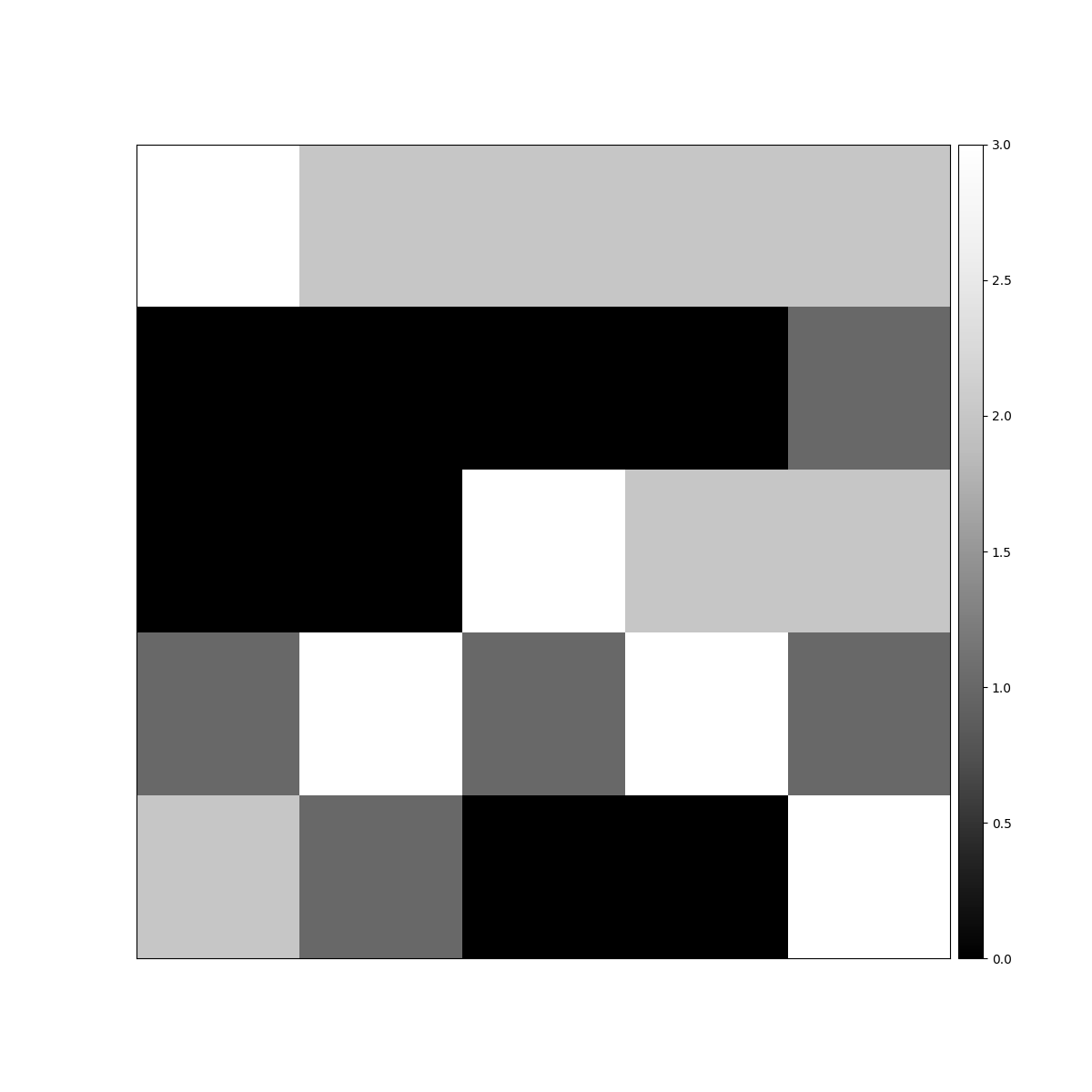Create Custom Discrete Legends with Earthpy

If you want to create a custom categorical legend, you can use the ep.draw_legend() function.

f, ax = plt.subplots(figsize=(8, 5))
im = ax.imshow(arr)
ep.draw_legend(im)
plt.tight_layout()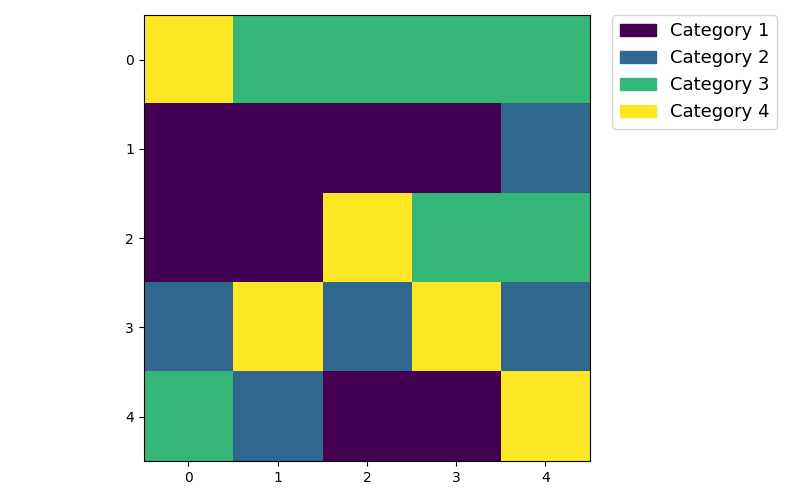Customize Discrete Legends

By default the draw_legend function creates a legend with default categories. You can customize the legend by adding titles.

f, ax = plt.subplots(figsize=(8, 5))
im = ax.imshow(arr)
ep.draw_legend(im, titles=["Small", "Bigger", "Even Bigger", "Ginormous"])
plt.tight_layout()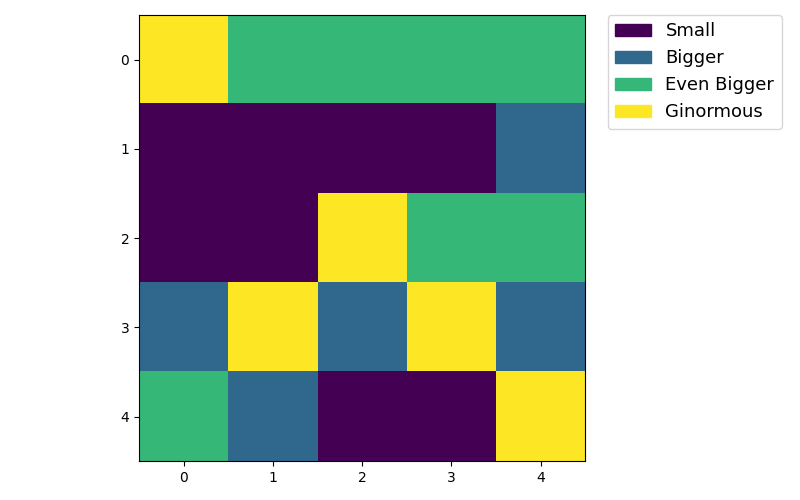Discrete Legends With Missing Values in the Array

Now let’s pretend that you are creating a series of classified plots. You may have a range of values that are expected between 0-4. However not all of your data has all values In this case, your legend won’t be able to by default create 4 categories because one doesn’t exist in your data. In this instance, you can specify the values explicitly:

new_arr = arr.copy()
new_arr[new_arr == 0] = 1

f, ax = plt.subplots(figsize=(8, 5))
im = ax.imshow(new_arr)
ep.draw_legend(
im,
titles=["Small", "Bigger", "Even Bigger", "Ginormous"],
classes=[0, 1, 2, 3],
)
plt.tight_layout()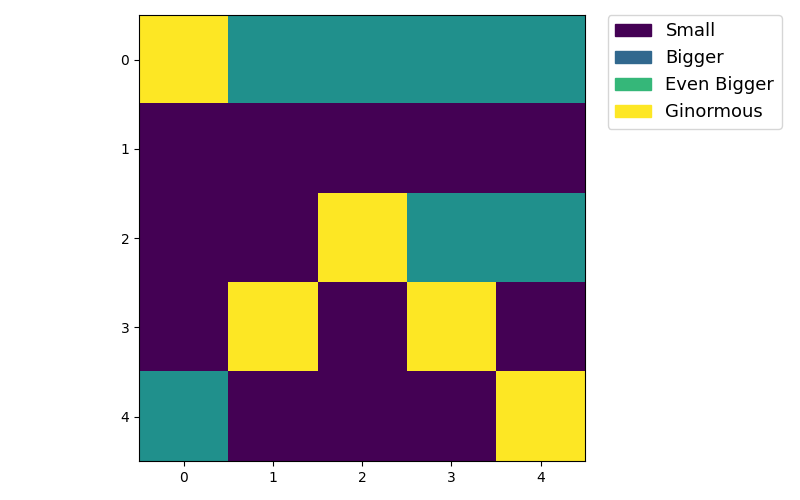Custom Colormaps and Ensuring Cmaps Apply to All Valid Classes

You can customize the color map used in your plot too. Notice that in this example, 4 category colors are rendered. Yet, the image only contains three values and thus will be rendered using three colors. The three colors used to render the image are incorrect by default. The colors begin at white and end at black even though the value of 0 which should be black is missing from the data.

f, ax = plt.subplots(figsize=(8, 5))
im = ax.imshow(new_arr, cmap="Greys_r")
ep.draw_legend(
im,
titles=["Small", "Bigger", "Even Bigger", "Ginormous"],
classes=[0, 1, 2, 3],
)
plt.tight_layout()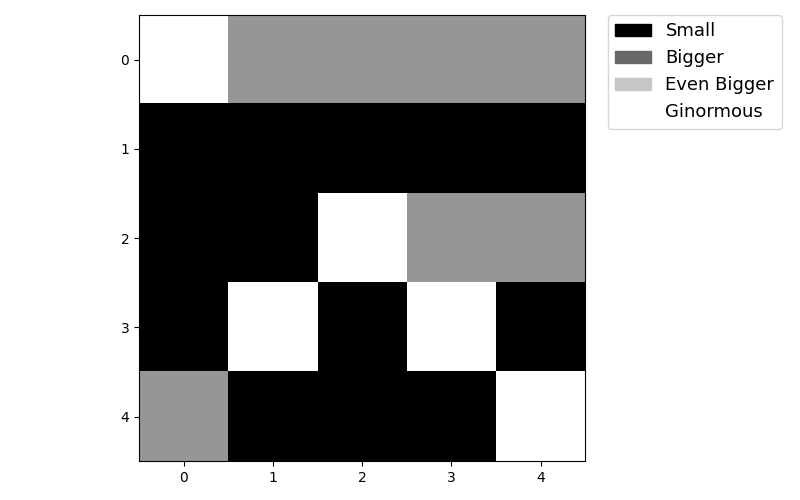Specify vmin and vmax to set the colormap range

In this case, you can use the vmin and vmax arguments to set the range of values to use for the colormap.

f, ax = plt.subplots(figsize=(8, 5))
im = ax.imshow(new_arr, cmap="Greys_r", vmin=0, vmax=3)
ep.draw_legend(
im,
titles=["Small", "Bigger", "Even Bigger", "Ginormous"],
classes=[0, 1, 2, 3],
)
plt.tight_layout()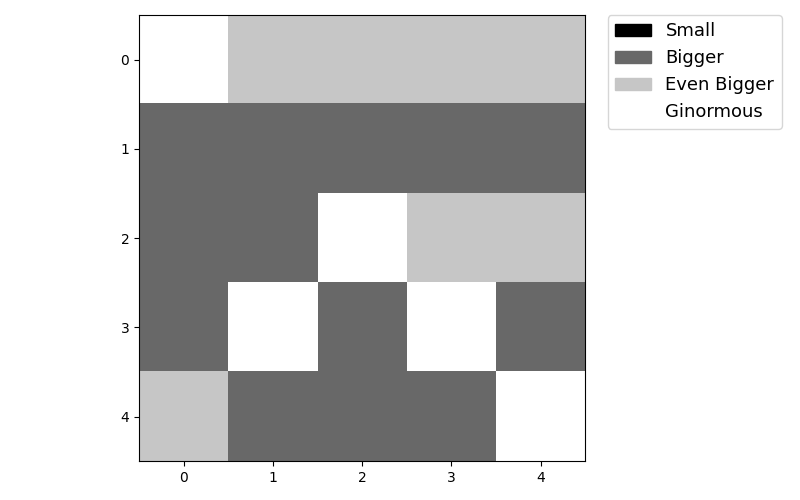Total running time of the script: ( 0 minutes 1.166 seconds)

Gallery generated by Sphinx-Gallery